# Arrays in JS

## Introduction

Like in any programming language, arrays in JS have a significant role in treating many variables as a single identity.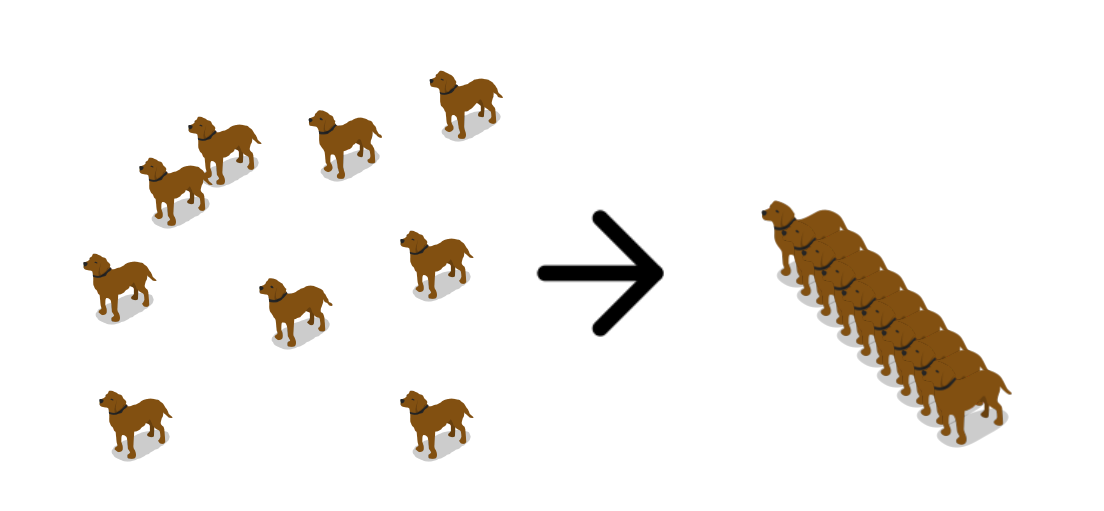This article will walk through the arrays, their operations using in-built functions.

Whatever this article covers might be easy for you to understand if you have prior knowledge of variables like const, let, var in javascript.

Ninja, shall we start now?Source: Giphy

## What are arrays in general?

An array data structure, or simply an array, is a data structure in computer science consisting of elements (values or variables), each of which is identified by at least one array index or key. Arrays are often used in many programming languages as they ease the work for programmers. Arrays assist programmers in writing beautiful and optimal code, but it also helps in memory management.

This is not it; there are several use cases of arrays that we can talk about later. Let us now have a look at what exactly are the arrays in js?

## Arrays in JS

Unlike C or C++, where an array is a variable or a data type, an array is an object in javascript.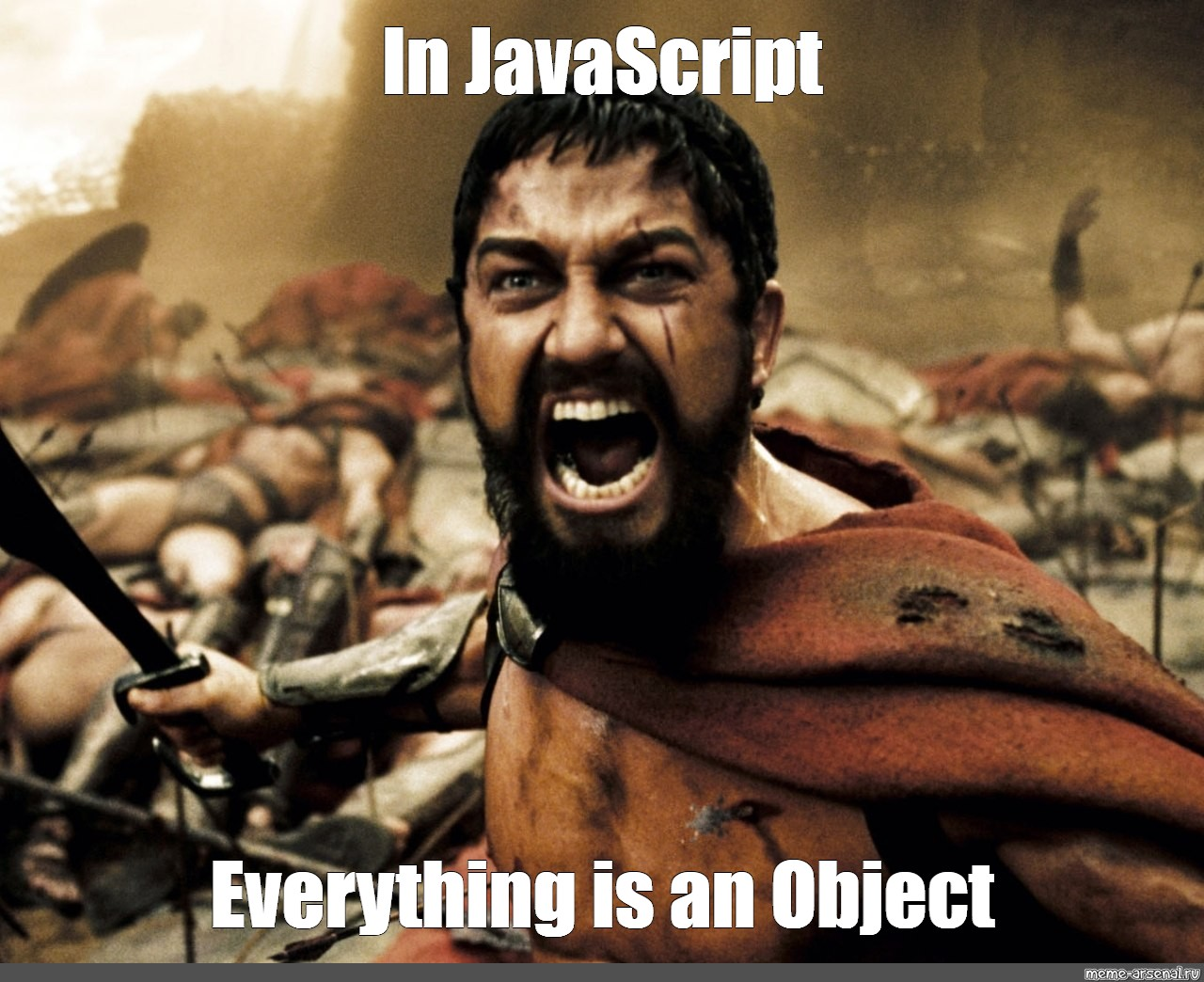Source: Meme

Well, that’s true; Objects in JavaScript are described as an unordered collection of connected data, of primitive or reference types, in the form of "key: value" pairs. In the context of an object, these keys can be variables or functions, referred to as properties and methods, respectively.

For example:

``````let school = {
name : "Ninjas School",
location : "Delhi",
established : "2001"
}``````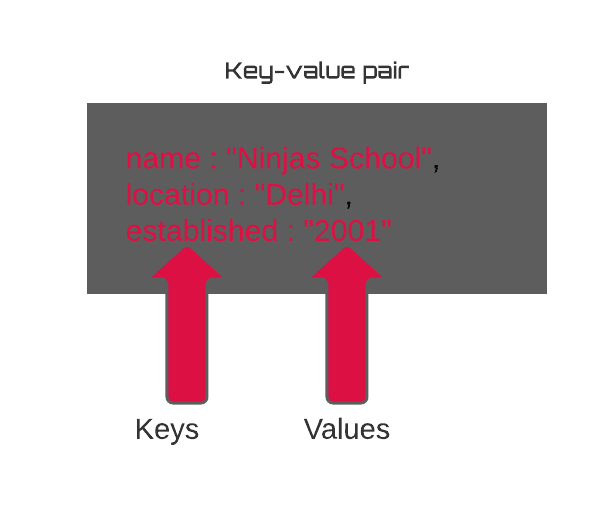You can store keyed collections of values in objects. That's all right.

However, we often require an ordered collection, with first, second, third elements, and so on.

For example, we might want to use it to keep track of a list of users, employees, faculty, and so on.

It is not practical to utilise an object since it lacks methods for managing element order. We can't add a new property "between" the ones that already exist. Objects aren't designed to be used in this way.

To hold ordered collections, there is a particular data structure called Array.

### Declaration

There are two syntaxes for creating an empty array:-

1. Using in-built function array():-
``let arr = new Array();``

2. Using conventional way:-

``let arr = [];``

Most of the time, the second syntax is used to declare an array object in javascript.

For example:-

``let lang = ["HTML", "CSS", "JS"];``

Let us discuss some properties of arrays in JS:

• Since an array is a javascript object, it can have heterogeneous values. Unlike in other languages, where arrays are limited to have the same type of items, such as integers, characters, strings, and so on, we can store any type of element in javascript. Example:

``const arr = ["Ninja", 1 , true];``

• Array indexes are zero-based: The first element in the array is 0, the second is 1, and so on.

For example: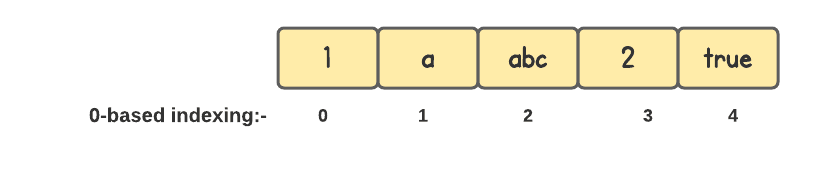### Accessing the elements

The elements of an array are numbered, starting with zero. We can get an element by its number in square brackets:

``````const planets = ["Venus", "Earth", "Mars"];
console.log( planets ); // Venus
console.log( planets ); // Earth
console.log( planets ); // Mars``````

• We can also use the element's index to replace it with another element.

``````planets = 'Saturn'; // now ["Venus", "Earth", "Saturn"]
console.log( planets ); // Venus
console.log( planets ); // Earth
console.log( planets ); // Saturn``````

• ...or add a new one to the collection:

``````planets = 'Mercury'; // now ["Venus", "Earth", "Saturn", “Mercury”]
console.log( planets ); // Venus
console.log( planets ); // Earth
console.log( planets ); // Saturn
console.log( planets ); // Mercury``````

• If we want to find the total number of elements in an array, we can use the length property:

``````console.log(planets.length); //4
// When we alter the array, the length property automatically updates. More specifically, it is the maximum numeric index plus one, not the number of values in the array.``````

Accessing each element manually is not a good way to walk through the elements. To enhance this, loops are introduced. A loop is a set of instructions that is repeatedly executed until a given condition is met.

### Looping array elements

for loop is one approach to walk through an array:

``````const os = ["Windows", "macOS", "Linux"];

for (let i = 0; i < os.length; i++) {
// one by one traversing over each element
console.log(os[i]);
}``````

Output

``````Windows
macOS
Linux``````

However, there is another type of loop for arrays, for..of:

``````const os = ["Windows", "MacOS", "Linux"];

// for..of loop
for (let type of os) {
console.log(type);
}``````

Output

``````Windows
macOS
Linux``````

Since arrays are objects, it is also technically feasible to use for..in:

``````const os = ["Windows", "macOS", "Linux"];

// for..in loop
for (let type in os) {
console.log(os[type]);
// Windows
// macOS
// Linux
}``````

However, this is a bad idea. It may have the following drawbacks:

• The for..in loop iterates through all properties, not just numerical ones.
• In the browser and other environments, there exist so-called "array-like" objects that resemble arrays. That is, they have length and index properties, but they may also have non-numeric properties and methods that we don't use very often. The for..in loop, on the other hand, will list them. These "additional" characteristics can become a problem if we need to interact with array-like objects.

In general, we should avoid using for..in with arrays.

Now that we've covered the fundamentals let's look at how to manipulate the array elements:

### Insertion and Deletion

Insertion refers to inserting one or more data elements into an array. A new element can be appended at the beginning, end, or any given index of an array based on the requirement.

Deletion is the process of eliminating an existing element from an array and reorganising all of its elements.

Javascript offers two functions to insert and delete the element at the beginning and the end.

Before we discuss the methods, let us look at the behaviour of the array in javascript.

Arrays in JavaScript can behave as both a queue and a stack. They allow you to add/remove elements from the beginning or the end.

Let us understand why?

A queue is one of the most common applications for an array. In computer science, this refers to an ordered set of elements that can perform two operations:

Push: appends an element to the end of a series.

Shift: get an element from the beginning of the queue, forwarding it so that the second element becomes the first.

Another application for arrays is the data structure known as a stack.

It supports two operations:

Push adds an element to the end, and pop removes an element from the end.

Pop:  element is taken from the end.  As a result, new elements are constantly added or taken from the "end."

Arrays support all the operations.

Methods that work with the end of the array:

push: append the element at the end of the array.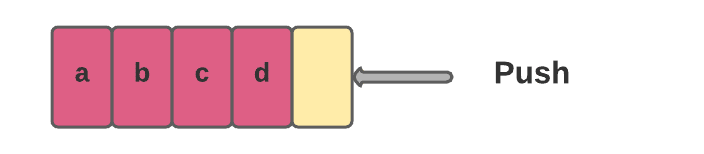``````const fruits = ["Apple", "Orange"];

console.log(fruits); // Apple, Orange
// push method to append the element at the end of the array
fruits.push("Pear");

console.log(fruits) // Apple, Orange, Pear``````

This call fruits.push(...).. is equal to fruits[fruits.length].

Output

``````[ 'Apple', 'Orange' ]
[ 'Apple', 'Orange', 'Pear' ]``````

pop: removes the element from the end of the array.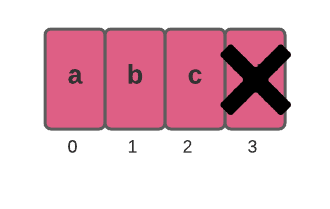``````const fruits = ["Apple", "Orange", "Pear"];
console.log( fruits );// Apple, Orange, Pear

console.log( "Removed element: " + fruits.pop() ); // remove "Pear"

console.log( fruits ); // Apple, Orange``````

Output

``````[ 'Apple', 'Orange', 'Pear' ]
Removed element: Pear
[ 'Apple', 'Orange' ]``````

Methods that work with the beginning of the array:

shift: Removes the first element of the array and returns it: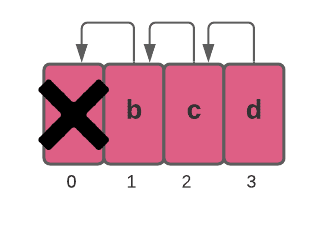``````const fruits = ["Apple", "Orange", "Pear"];

console.log( fruits ); // Apple, Orange, Pear

console.log("Removed element: " + fruits.shift() ); // remove Apple

console.log( fruits ); // Orange, Pear``````

Output

``````[ 'Apple', 'Orange', 'Pear' ]
Removed element: Apple
[ 'Orange', 'Pear' ]``````

unshift: Add the element at the beginning of the array and returns it: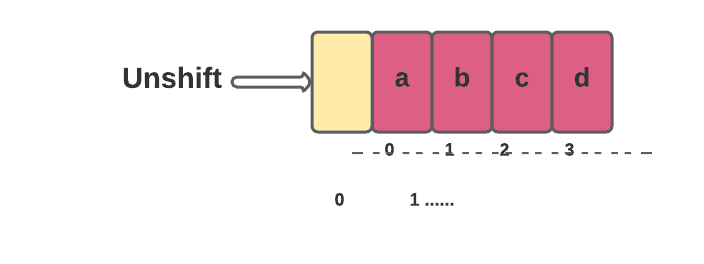``````const fruits = ["Orange", "Pear"];

console.log( fruits ); // Orange, Pear

fruits.unshift('Apple');

console.log( fruits ); // Apple, Orange, Pear``````

Output

``````[ 'Orange', 'Pear' ]
[ 'Apple', 'Orange', 'Pear' ]``````

Let's look at the performance of each of the methods we've examined so far: -

Which method, in your opinion, must have poor performance?

Those who are working at the start of the array, of course.

Let us try to figure out why.

Push/pop methods are fast, whereas shift/unshift methods are slow.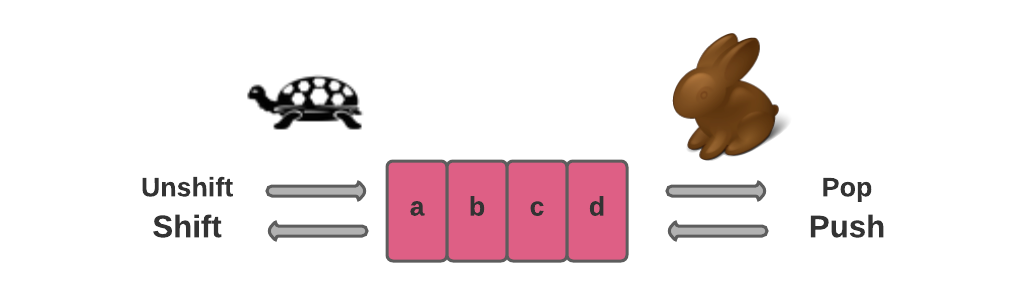Why is working with the end of an array faster than working with the beginning? Let's take a look at what occurs during the execution:

``fruits.shift(); // take 1 element from the start``

The shift method must do three things:

• Remove the element with the 0-index.
• All elements should be moved to the left and renumbered from 1 to 0, 2 to 1, and so on.
• Make a change to the length property.

The larger the array, the longer it takes to move the elements, requiring more in-memory operations.

Unshift works in a similar way: to add an element to the beginning of an array, we must first move existing elements to the right and increase their indexes.

What's the deal with push/pop? There is no need for them to relocate anything. The pop method cleans the index and shortens the length to extract an element from the end.

### Searching

Javascript has four built-in functions for searching a specific element in an array, which vary based on the use case:

The use cases could be:

Do you want to get all of the elements in an array that satisfy a certain criterion?

Do you wish to check if any of the elements fulfill the criteria?

Do you want to see if a particular value is present in the array?

Or are you looking for the index of a value in an array?Source: meme

This section covers all the use cases:-

Searching methods are:-

• Filter
• Find
• Includes
• IndexOf

Let us discuss them.

#### Array.filter()

To find elements in an array that fulfill a specific condition, we can use the Array.filter() method.

Syntax:

``````const array_name = array.filter(callback);
// callback is the callback function that is applied to each element of the array.``````

For instance, if we want to get all positive items in an array of numbers, we can do this:

``````const array = [10, -20, -3, 30, -50];

const positiveNum = array.filter(element => element > 0);

console.log(positiveNum) //[10, 30]``````

If no elements meet the applied condition, an empty array is returned.

There are situations when we don't require all of the elements that satisfy a specific requirement. We only need one element that fulfills the criterion. In that scenario, the find() method is required.

#### Array.find( )

To identify the first element that meets a given requirement, we utilise the Array.find() method. It takes a callback as a parameter and returns the first element that satisfies the callback criteria, just as the filter method.

Syntax:-

``const element = array.find(callback);``

Let us take the previous example:-

``````const array = [10, -20, -3, 30, -50];

const positiveNum = array.find(element => element > 0);

console.log(positiveNum )//10``````

It's worth noting, though, that if no item in the array matches the criterion, the result is undefined.

What if you want to see if a specific element is in an array? How do you go about doing this?

#### Array.includes( )

The includes() method checks whether an array contains a given value and returns true or false depending on the result.

Syntax:-

``````const element = array.includes(valueToFind, fromIndex)
// valueTOFind is the parameter to check for the specific element
// fromIndex(optional) is the starting index from where you want to start searching``````

So, in the example above, if we want to see if 20 is one of the array's items, we can do so as follows:

``````const array = [10, -20, -3, 30, -50];

const ele= array.includes(20);

console.log(ele) // false``````

#### Array.indexOf( )

The indexOf() method returns the first index in an array where a specific element can be found. If the element does not exist in the array, it returns -1.
Syntax:-

``````const indexOfElement = array.indexOf(element, fromIndex)
// element is the element we are checking for in the array (required), and
// fromIndex is the index in the array that you want to start searching for the element from (optional)``````

Let us try to find the index of the element 10:-

``````const array = [10, -20, -3, 30, -50];

const indexOfTwo = array.indexOf(10);

console.log(indexOfTwo) // 0``````

It's worth noting that both the includes and indexOf methods search the array with strict equality('==='). They'll return false and -1 if the values are of different types (for example, '2' and 2).

1. How much size each element of array takes in memory?
Each array element in a different array type will require a different number of bytes to be stored.
For example,
Each array element in a byte (typed) array is stored in 1 byte.
Each array element in a short (typed) array is stored in two bytes.
Each array element in an int (typed) array is stored in 4 bytes.
2. How is an array stored in memory?
The elements of an array are stored contiguously in memory.
3. What are the dynamic arrays in javascript?
In JavaScript, a dynamic array indicates that the size of the array is automatically increased or decreased. Because JavaScript is not type-dependent, there is no such thing as a static array. When an element is deleted from an array, the size of the array must be reduced, and when an element is added to an array, the size of the array must be increased.

## Key takeaways

To summarise the session, we looked at the arrays in JS as well as fundamental operations utilising built-in functions. It's now time to put each program into action and modify it. If necessary, go over each strategy again and put it into practise on your desk to obtain a deeper understanding.

Don’t stop here Ninja, keep exploring.

Furthermore, we have a variety of well-structured courses just for you. So, what are you waiting for to get yourself enrolled now?